# 10 By 10 Grids Printable

PDF files are available
Updated: Jun 02, 2021
Author:
Editor:

Number grids are square grids worksheets that have rows and columns with numbers arranged in them. Well, all the numbers in the row and column have a relationship with each other.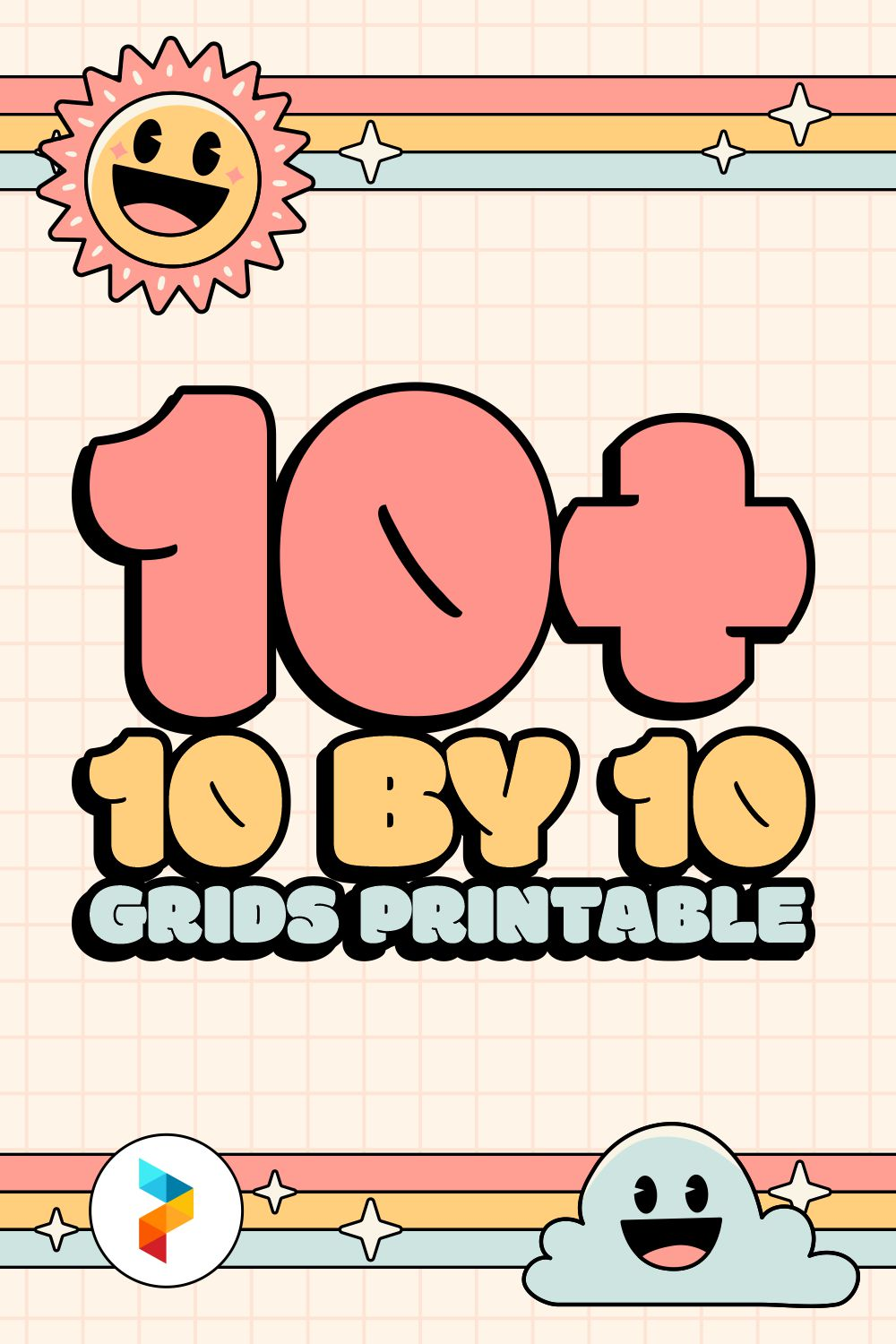### Table of Images 👆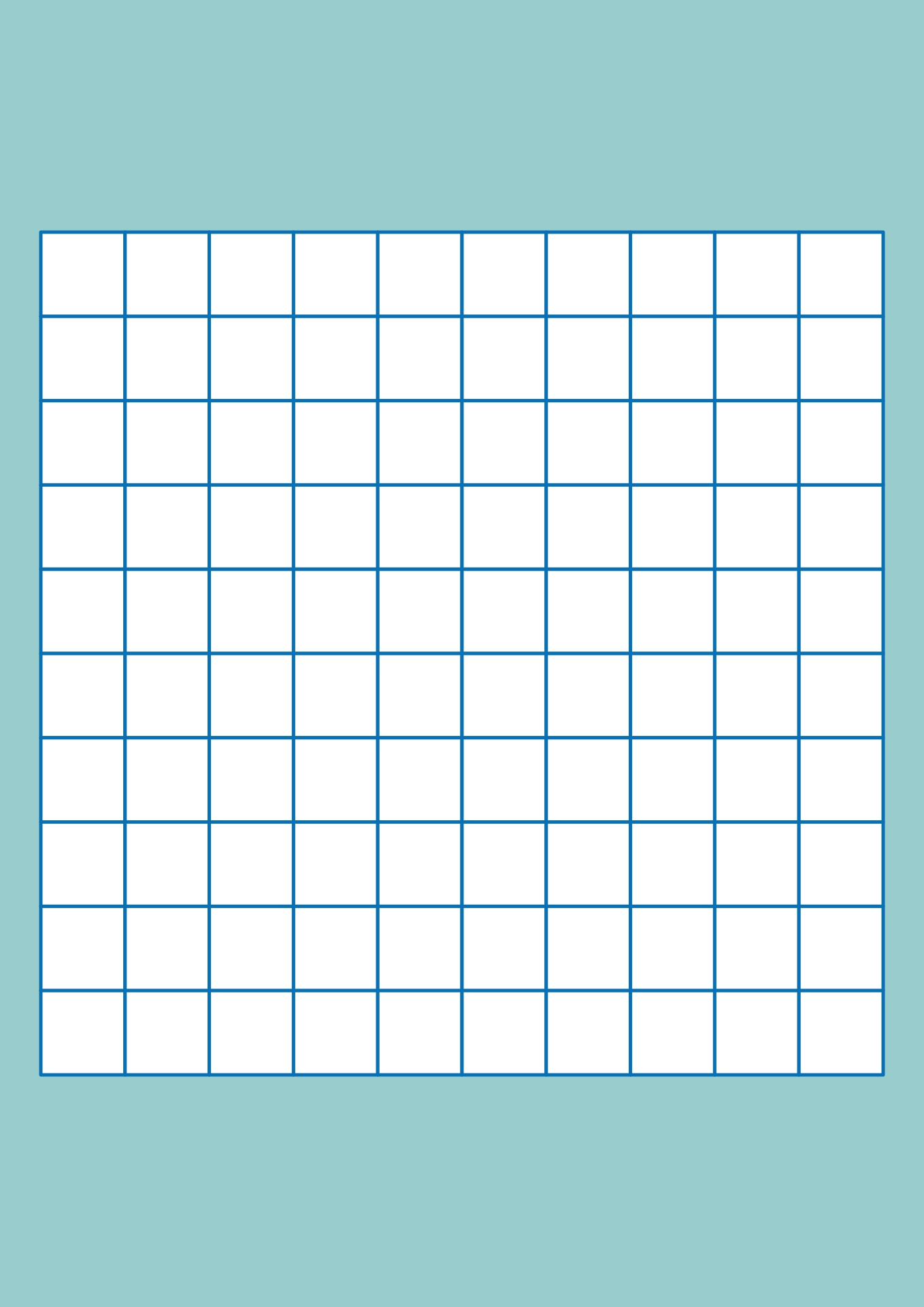## What is a Number Grid?

Number grids are square grids worksheets that have rows and columns with numbers arranged in them. Well, all the numbers in the row and column have a relationship with each other. So, actually, the main concept of the number grid is to check the relationship between the numbers in the column and row.

The number grid has an empty square. Now, you have to fill in the empty area according to the pattern of the column and row relationships. That's what makes the number grid help everyone to do math calculations well.

### What are 10 By 10 Grids?

Among the various types of number grids, 10 by 10 grids are one of the most widely used number grids, especially for children's learning. It is a grid that has a 10 x 10 grid with the numbers 1-100. However, not all squares contain numbers.

There are several empty boxes. Now, the blanks must be filled in with a certain number (in the range of 1-100) according to the row and column relationship patterns.

The 10 by 10 grids can be used not only for learning math but also for various other purposes such as word games and making designs. However, there are no numbers on the 10 by 10 grid for games and designs. So, it is a blank 10 by 10 grid.

## How to Use a 10 By 10 Grid to Learn Math?

Because 10 by 10 grids are so useful, you can teach children to use 10 by 10 grids. So, let’s talk about how to use it to learn math here.

1. ### Calculating and Understanding Place Value in Numbers

First, the 10 by 10 grid can be used to calculate large numbers. Ask them to calculate the two numbers on the 10 by 10 grid. An example is the numbers 1-50 and 25. Then, the results are used to learn the place value. With this one activity, children can learn two important concepts in mathematics.

2. ### Numbers Puzzle

The next method to use the 10 by 10 grid as a math lesson is to ask the children to write numbers in the empty boxes on the 10 by 10 grid. An example is the numbers between 10 and 15.

3. ### Analyzing Number Patterns

You can also teach children to look for patterns of each number in the rows and columns of the 10 by 10-grid. First, ask the children to count each number in a row and column. Then, they have to look for patterns in those numbers. After they find the pattern, they have to compare the pattern with other friends.

4. ### Calculating Multiples of Numbers

Teach children to calculate multiples of numbers with that 10 by 10 grid. This one activity will make children proficient in multiplication and division. The trick is to ask the children to count the multiples of each number in the 10 by 10 grid.

5. ### Finding Odd and Even Numbers

You can also use the 10 by 10 grid as a tool to teach kids about even and odd numbers. You can also teach kids about prime numbers using the 10 by 10 grid. All even and odd numbers can be searched in the columns and rows of the 10 by 10 grid.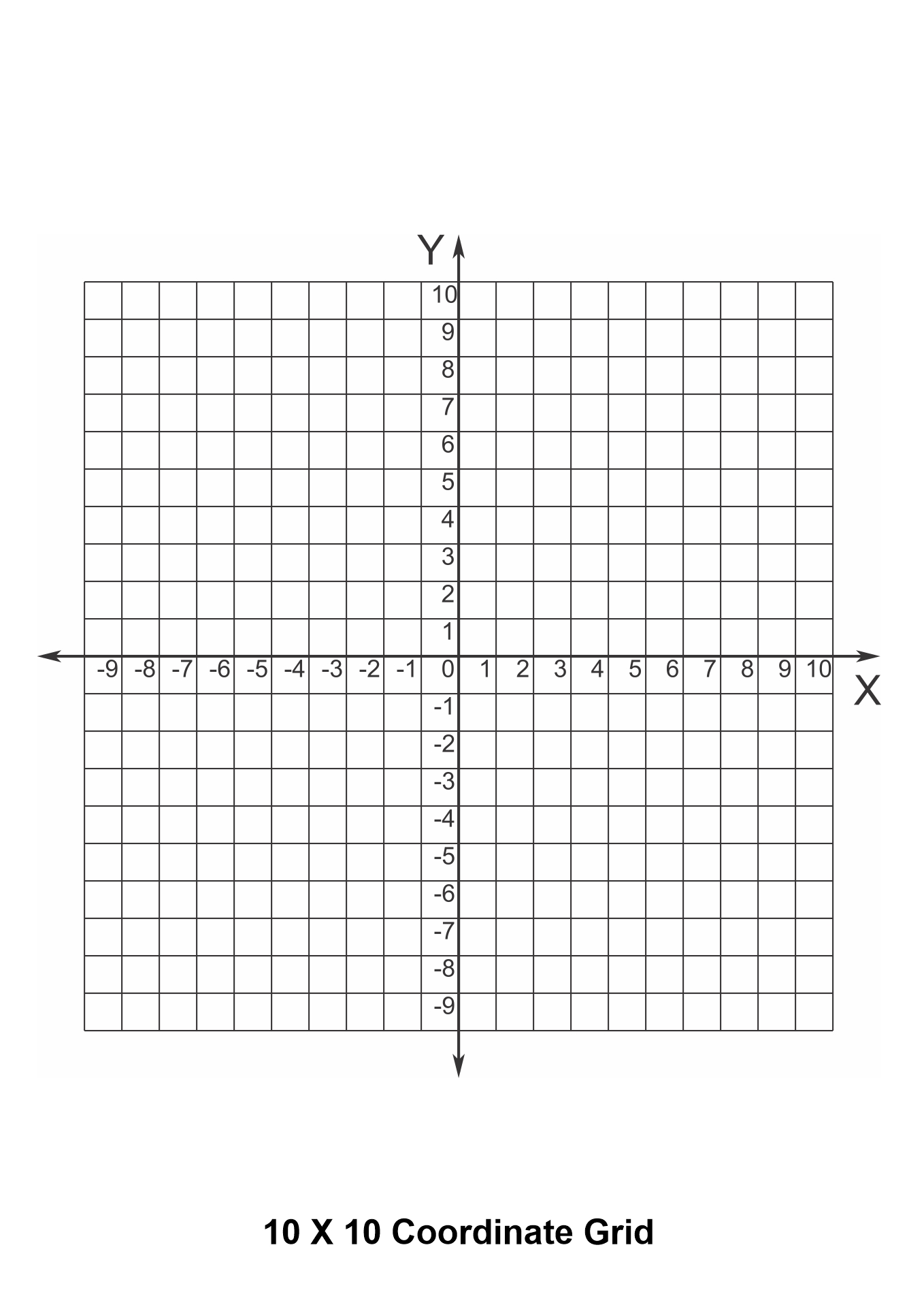We also have more printable other you may like:
Printable Pumpkin Seed Recipe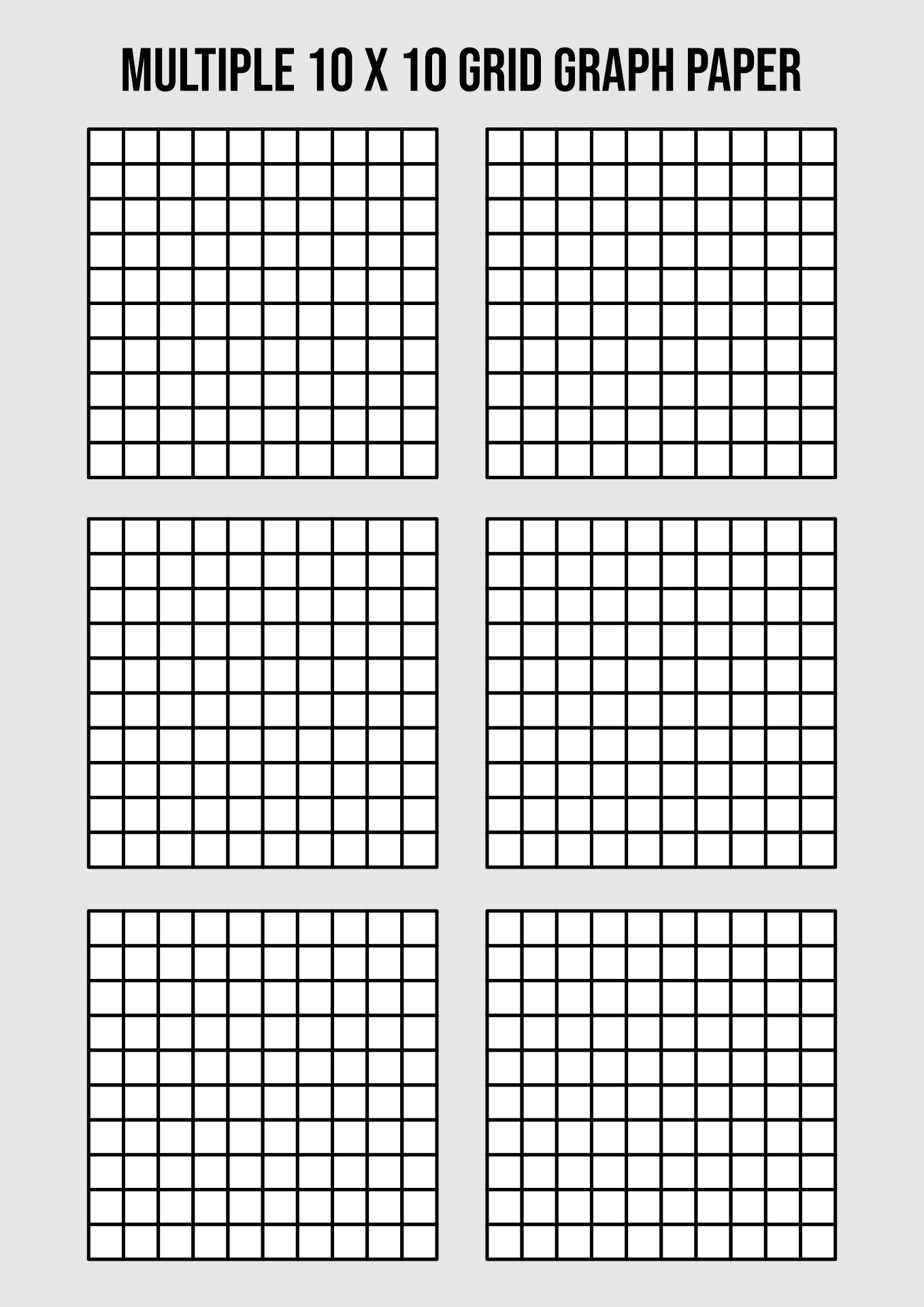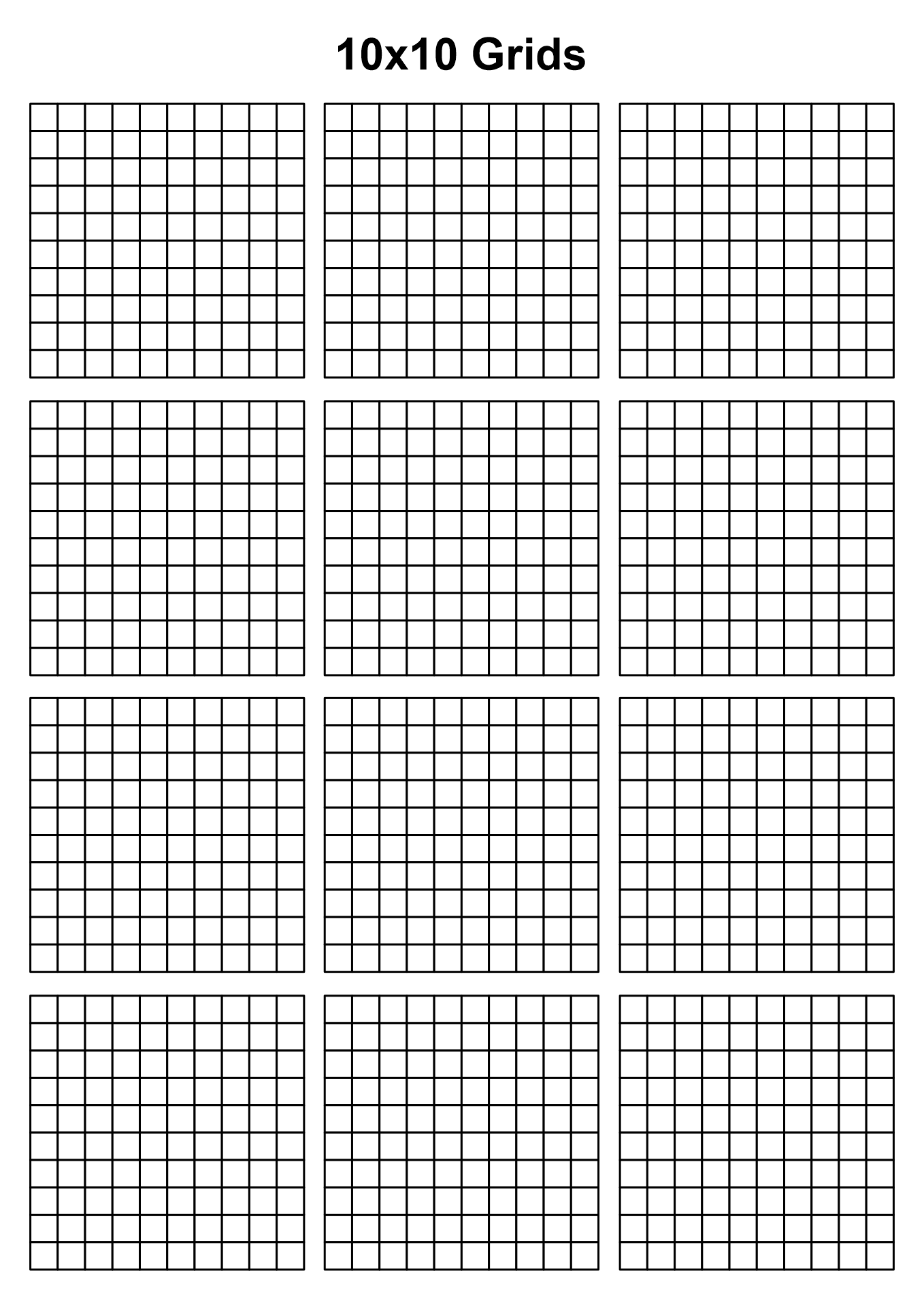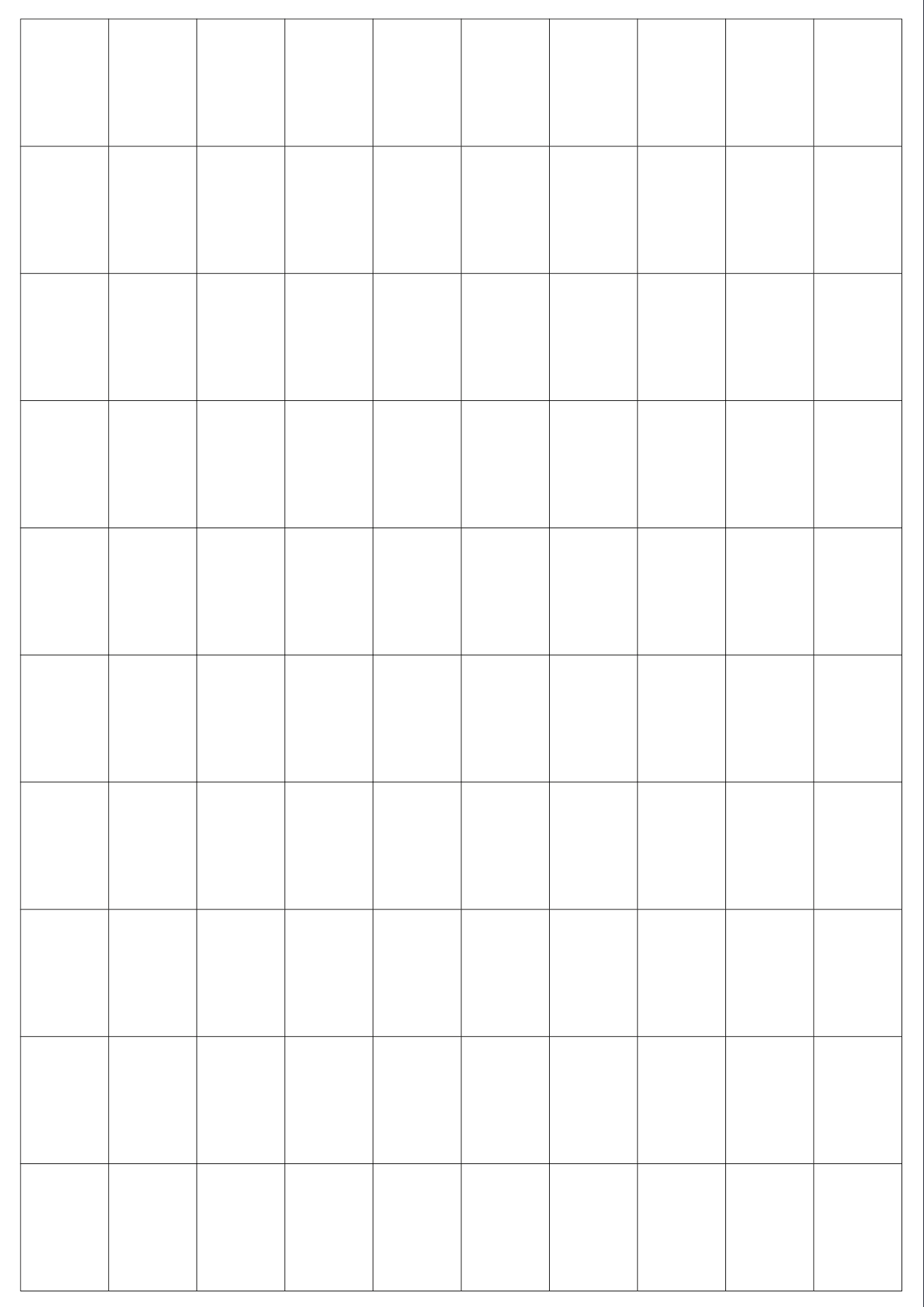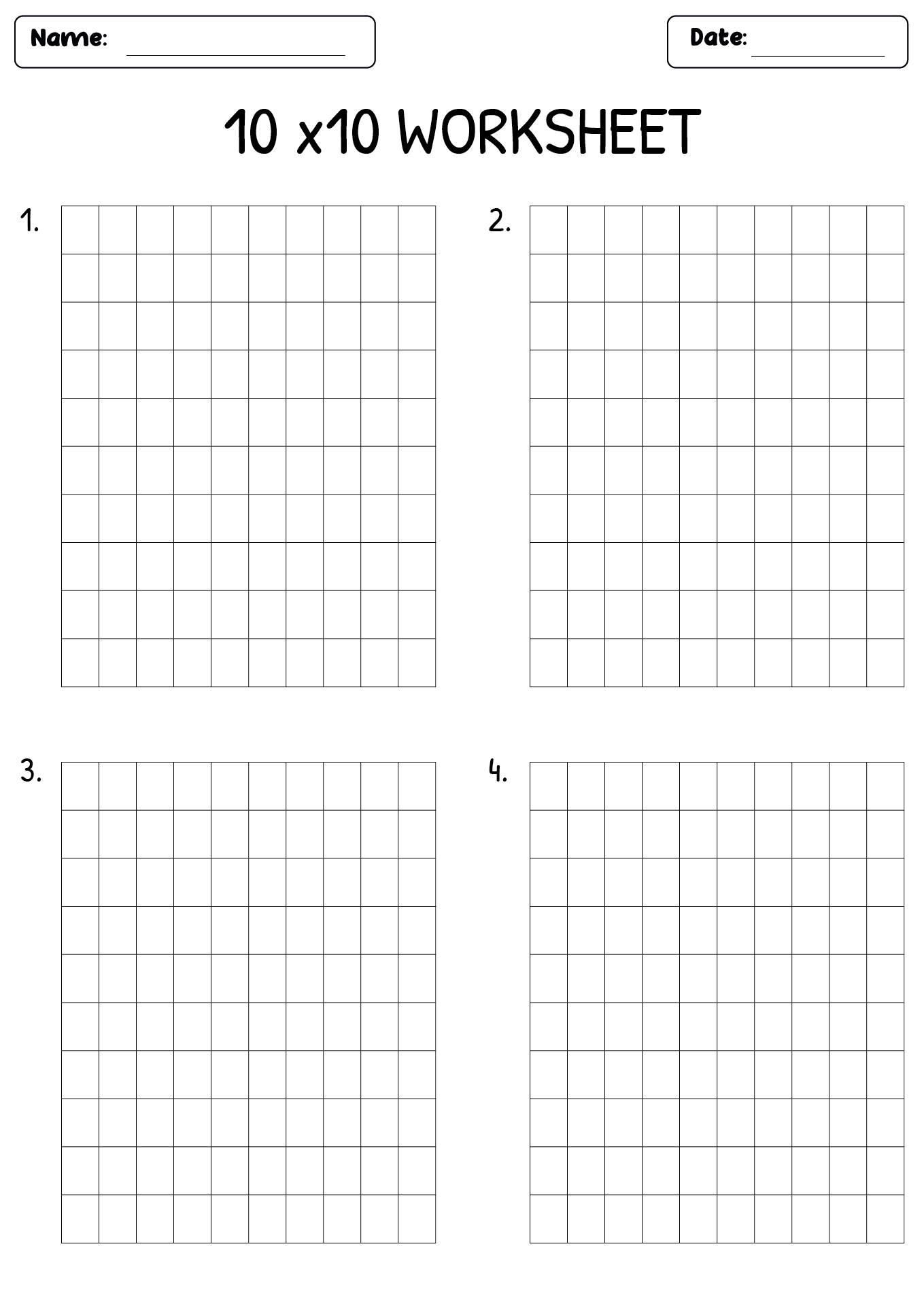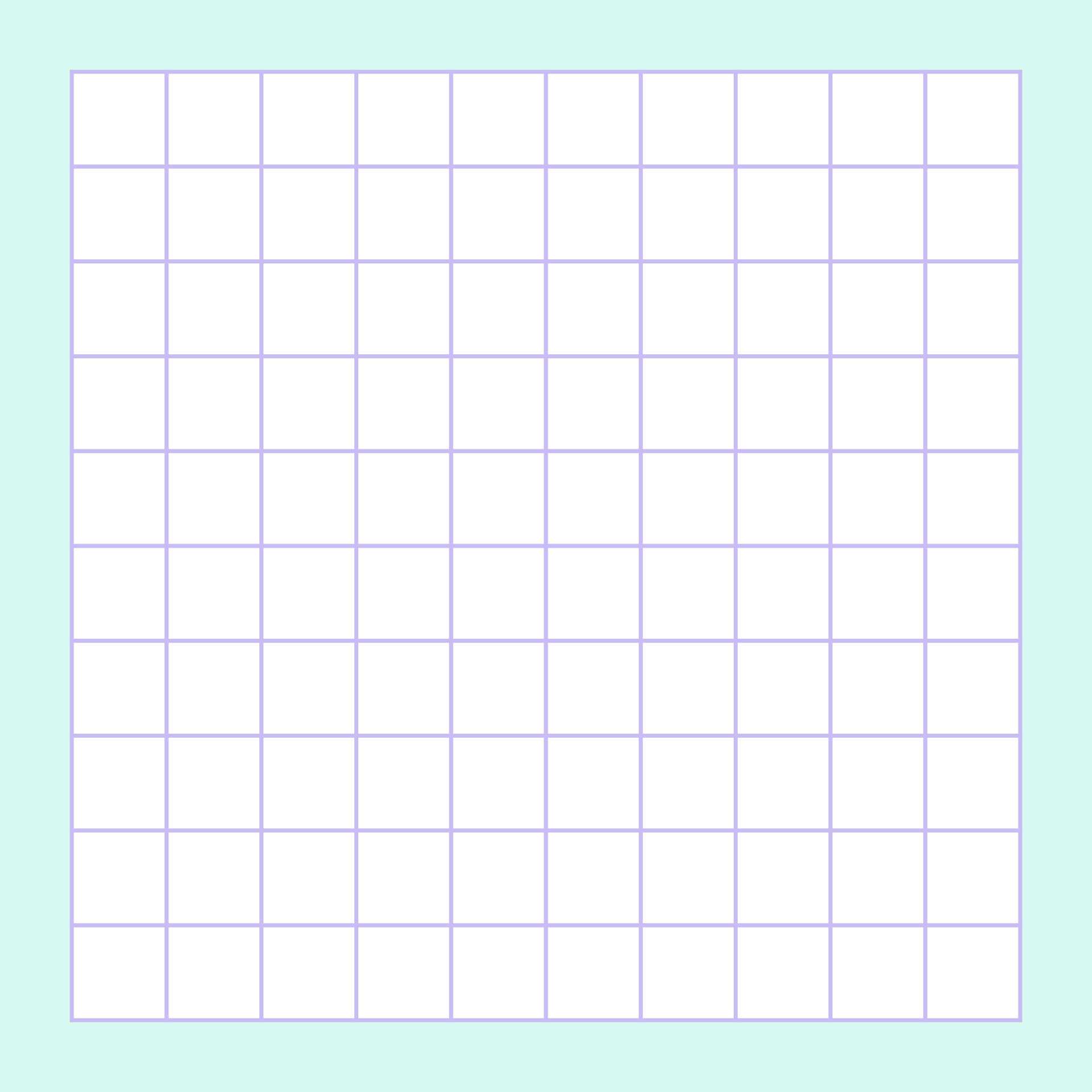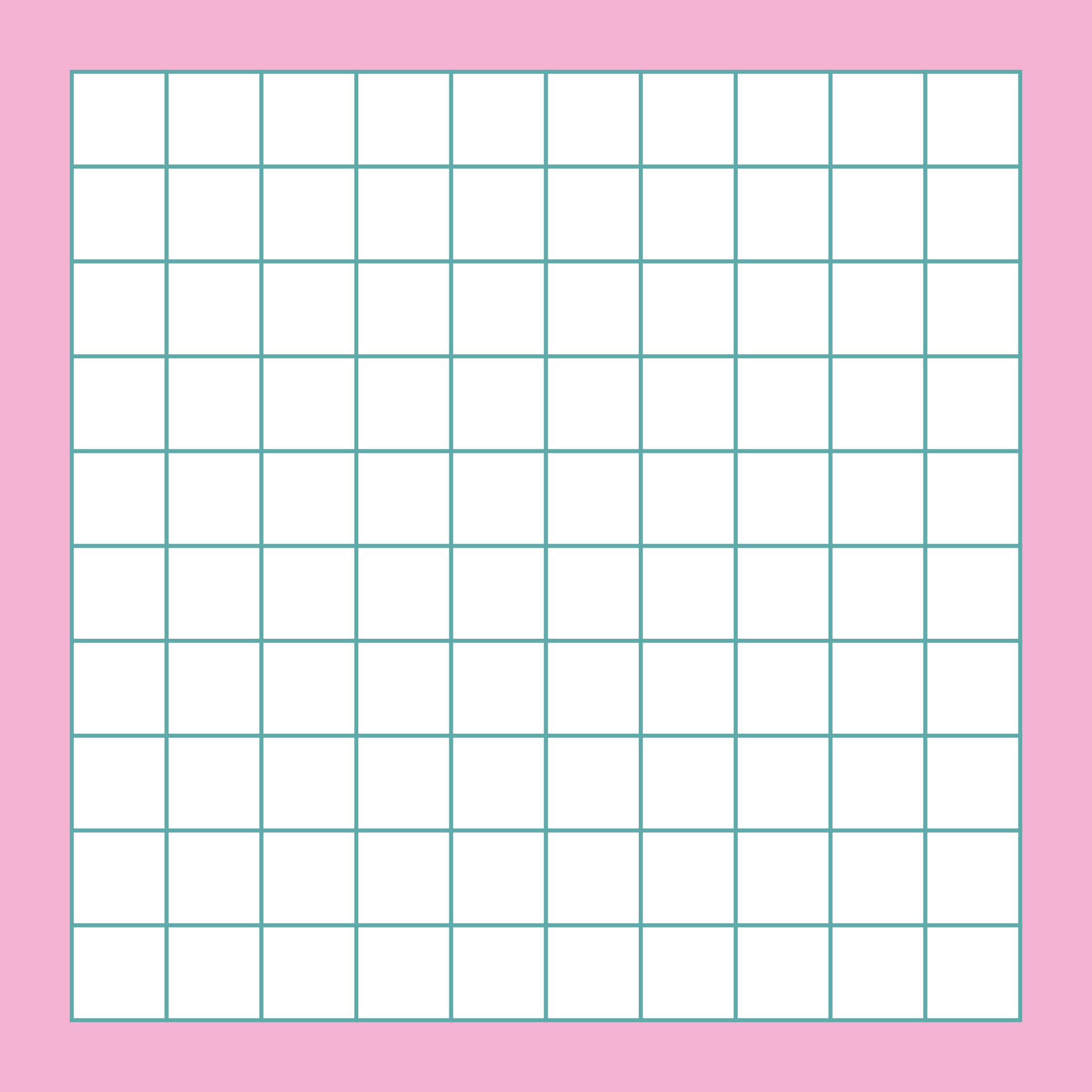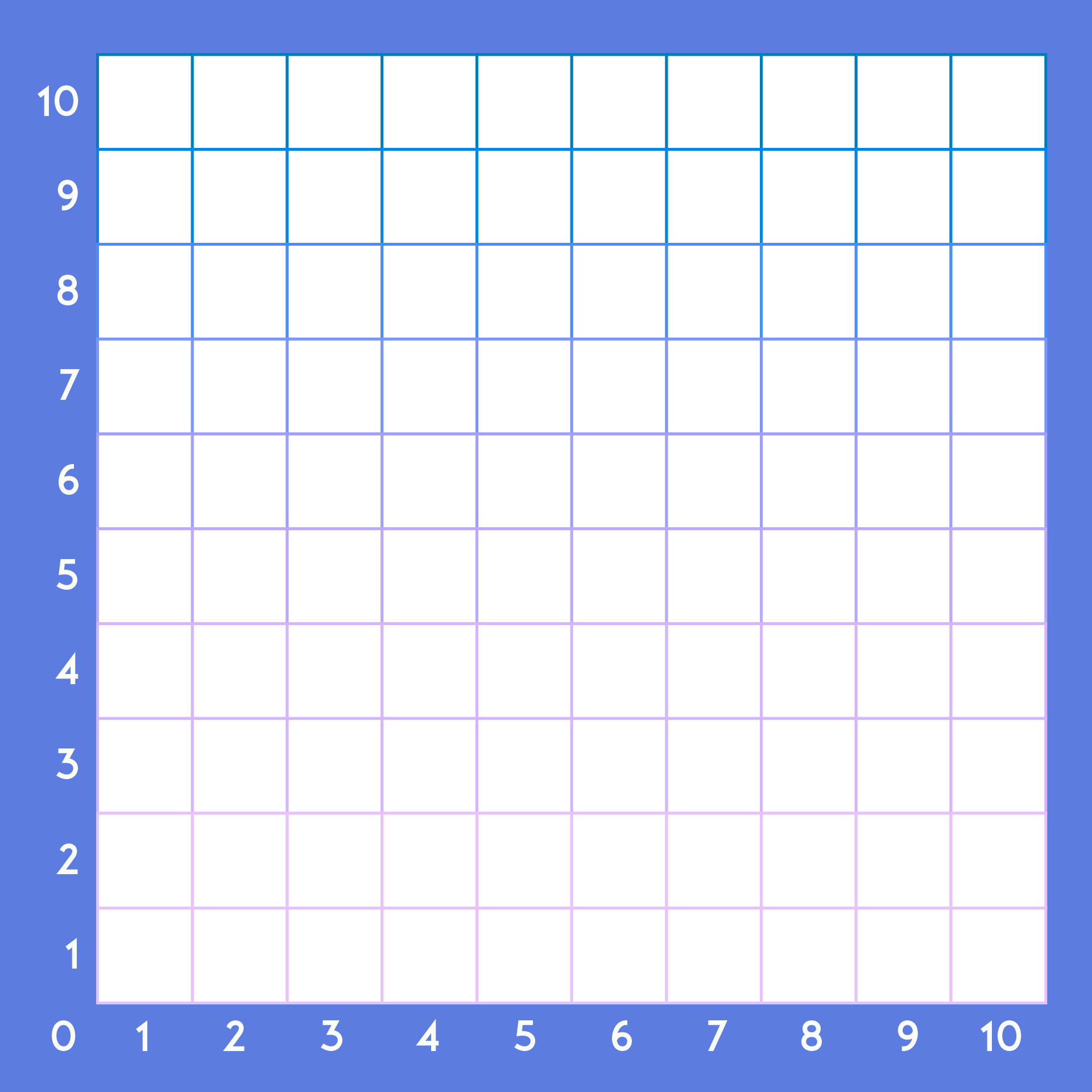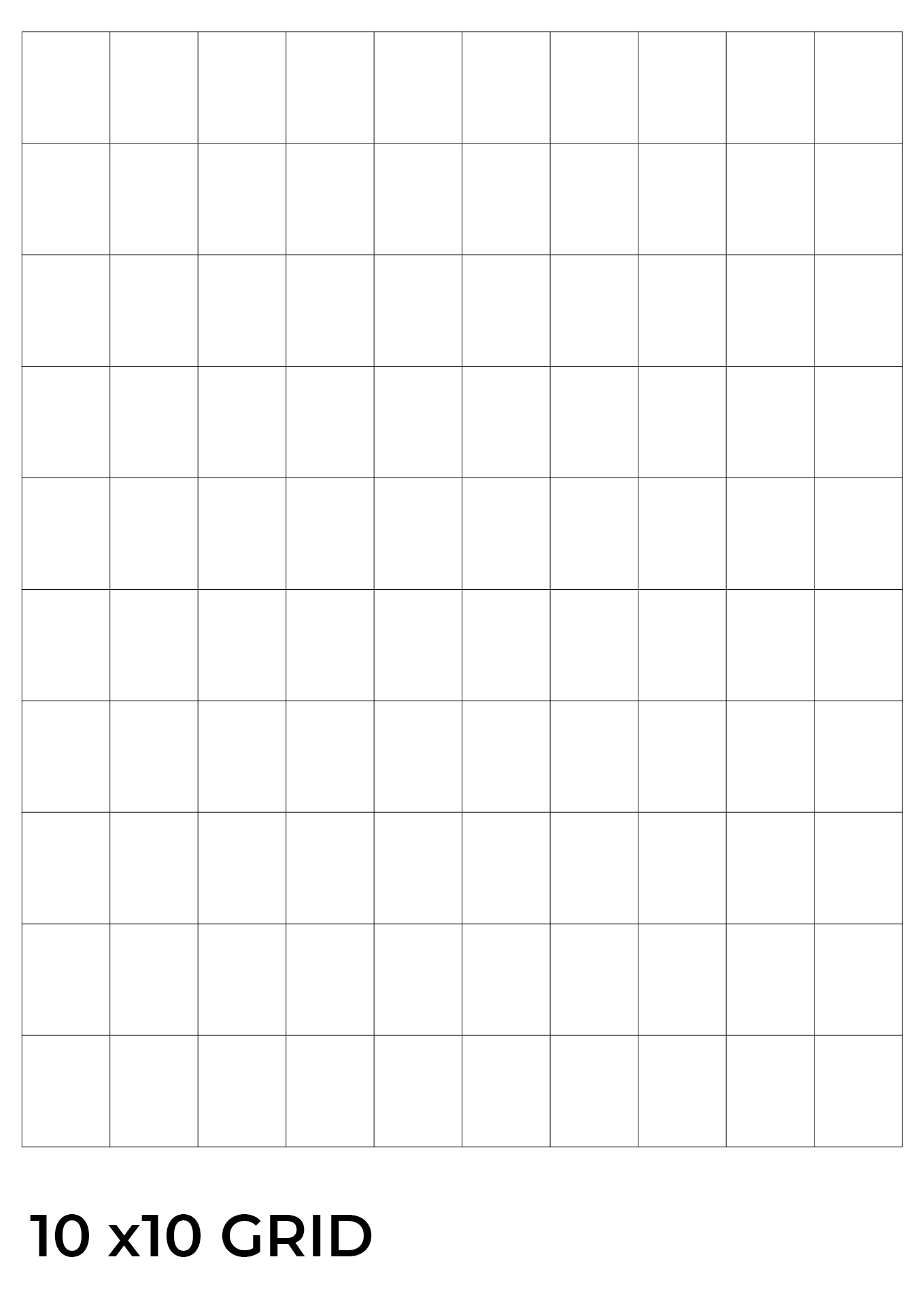## How to Teach Kids about 10 By 10 Grids?

Teaching children about the 10 by 10 grid is not easy, so you need to do these strategies so children can easily understand the use of the 10 by 10 grid.

• You have to help children to develop their number sense first. Give them a grid graph paper. Don't write numbers on the 10 by 10 grid. Then, ask the children to write the numbers 1-100 on the 10 by 10 grid. Do that every day so the kids can memorize all the numbers.

• If you have done the first method above, now you can use a big 10 by 10 grid and place it on the wall. Then, use the magnetic numbers to play the 10 by 10 grid. Teach children about simple calculations using the 10 by 10 grid. They have to fill in the blanks with the calculation results.

• Ask the children to color each of the squares with a different color. You can also ask them to give two different colors to even and odd numbers.

• Divide the children into several groups. Then, each group should make a 10 by 10 grid. You can also give quizzes that must be completed by all teams. Give them questions about simple math operations using a 10 by 10 grid.

• Among the various arithmetic operations, the division is one of the most difficult calculations for children. Therefore, you can teach division to children using a 10 by 10 grid. Draw numbers on the 10 by 10 grid diagonally. Then, ask the children to do a division calculation using those numbers.

• Use one dice and each child must play the dice. An example is a child rolling a dice 2 times. The dice show the numbers 2 and 3. So, the child must calculate the multiplication of the numbers in column 2 and row 3.

Article written by Shasmitha Mutiara, last updated on Jun 02, 2021 and edited by Printablee Team.

#### More printable images tagged with:

Have something to tell us?

1.Emma

I found the 10 by 10 Grids Printable incredibly helpful for organizing my information. It's simple yet effective.

2.Ingrid

Printable images with 10 by 10 grids are practical tools for various activities and crafts, enabling precise measurements and easy organization.

3.Matthew

I found the 10 by 10 grids printable incredibly useful for organizing my thoughts and creating visually appealing designs. Thank you for this simple yet effective resource!

4.Nathan

I love the simplicity of these 10 by 10 grids! They are perfect for organizing my ideas and planning out projects. Thank you for providing such a useful printable resource!

5.Kate

This printable resource is a helpful tool for organizing data and visuals in a clean and efficient way. It simplifies complex concepts and enables better understanding.

6.Evan

I found the 10 by 10 grids printable resource extremely helpful for organizing my work and planning out projects. It's simple yet effective for tracking progress and brainstorming ideas. Highly recommended!

7.Sloane

Printable images with 10 by 10 grids allow for easy organization and precise placement of elements, making them incredibly useful for designing graphs, charts, or puzzles.

8.Savvy

I find the 10 by 10 Grids Printable very helpful for organizing and visualizing information. It offers a simple yet effective tool for various projects.

9.Noah

This printable resource is a helpful tool for visualizing and organizing data in a structured manner. It's convenient and straightforward to use for various activities, making it a valuable addition to any learning or creative setting.

10.Thea

Printable images in 10 by 10 grids offer a convenient visual format for organizing and analyzing data, making it easier to spot patterns or identify specific elements within a larger image or dataset.